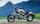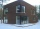The rectangular crossroads comes passenger car and an ambulance, the ambulance left. Passenger car is at 43 km/h and ambulance 52 km/h.

Calculate such a relative speed of the ambulance moves to the car.

Result

Relative speed of ambulance to car:  67.48 km/h

Solution:Leave us a comment of example and its solution (i.e. if it is still somewhat unclear...):Be the first to comment!To solve this example are needed these knowledge from mathematics:

Two vectors given by its magnitudes and by included angle can be added by our vector sum calculator. Pythagorean theorem is the base for the right triangle calculator.

Next similar examples:

1. Four ropesTV transmitter is anchored at a height of 44 meters by four ropes. Each rope is attached at a distance of 55 meters from the heel of the TV transmitter. Calculate how many meters of rope were used in the construction of the transmitter. At each attachment.
2. Chord circleThe circle to the (S, r = 8 cm) are different points A, B connected segment /AB/ = 12 cm. AB mark the middle of S'. Calculate |SS'|. Make the sketch.
3. Broken treeThe tree is broken at 4 meters above the ground and the top of the tree touches the ground at a distance of 5 from the trunk. Calculate the original height of the tree.
4. CablewayCableway has a length of 1800 m. The horizontal distance between the upper and lower cable car station is 1600 m. Calculate how much meters altitude is higher upper station than the base station.
5. Cars 6At 9:00 am two cars started from the same town and traveled at a rate of 35 miles per hour and the other car traveled at a rate of 40 miles per hour. After how many hours will the cars be 30 miles apart?
6. Round-tripA woman works at a law firm in city A which is about 50 miles from city B. She must go to the law library in city B to get a document. Find how long it takes her to drive​ round-trip if she averages 40 mph.The ladder has a length 3.5 meters. He is leaning against the wall so that his bottom end is 2 meters away from the wall. Determine the height of the ladder.
8. Broken treeThe tree was 35 meters high. The tree broke at a height of 10 m above the ground. Top but does not fall off it refuted on the ground. How far from the base of the tree lay its peak?
9. WindbreakA tree at a height of 3 meters broke in the windbreak. Its peak fell 4.5 m from the tree. How tall was the tree?
10. Trio 2Decide whether trio of numbers is the side of a right triangle: 26,24,10.
11. HeightIs right that in any right triangle height is less or equal half of the hypotenuse?
12. Vector 7Given vector OA(12,16) and vector OB(4,1). Find vector AB and vector |A|.
13. MinuteTwo boys started from one place. First went north at velocity 3 m/s and the second to the east with velocity 4 m/s. How far apart they are after minute?
14. Bus and MilanThe bus started from the village at speed 60 km/h. After one kilometer, when Milan came to bus stop on a motorcycle with grandmother. When they found that bus drove away he try to catch it. At the next stop, a distance of 3km caught up bus. How quickly had
15. Bob traveledBob traveled 20 meters to Dave's house. He arrived in 3 minutes. What was his average speed for the trip.
16. Plane IIA plane flew 50 km on a bearing 63degrees20 and the flew on a bearing 153degrees20 for 140km. Find the distance between the starting point and the ending point
17. GlovesI have a box with two hundred pieces of gloves in total, split into ten parcels of twenty pieces, and I sell three parcels. What percent of the total amount I sold?